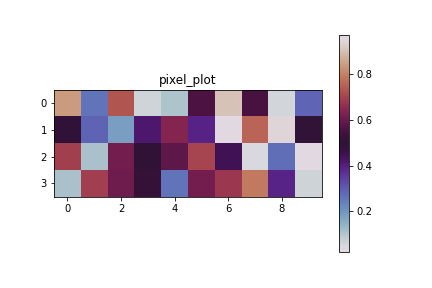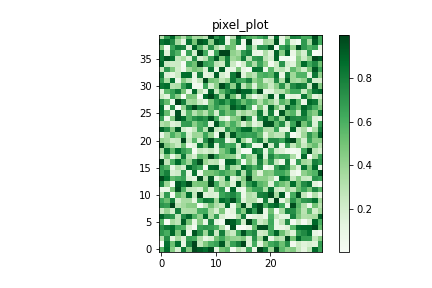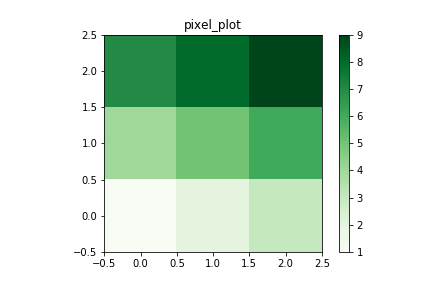Open in App
Not now

# Create 2D Pixel Plot in Python

• Last Updated : 08 May, 2021

Pixel plots are the representation of a 2-dimension data set. In these plots, each pixel refers to a different value in a data set. In this article, we will discuss how to generate 2D pixel plots from data. A pixel plot of raw data can be generated by using the cmap and interpolation parameters of the imshow() method in matplot.pyplot module.

### Syntax:

matplotlib.pyplot.imshow(X, cmap=None, norm=None, aspect=None, interpolation=None, alpha=None, vmin=None, vmax=None, origin=None, extent=None, shape=, filternorm=1, filterrad=4.0, imlim=, resample=None, url=None, \*, data=None, \*\*kwargs)

### Approach:

The basic steps to create 2D pixel plots in python using Matplotlib are as follows:

Step 1: Importing Required Libraries

We are importing NumPy library for creating a dataset and a ‘pyplot’ module from a matplotlib library for plotting pixel plots

```import numpy as np
import matplotlib.pyplot as plt```

Step 2: Preparing data

For plotting, we need 2-dimensional data. Let’s create a 2d array using the random method in NumPy. Here data1 array is of three sub arrays with no of elements equal to 7, while data2 is an array of four sub-arrays with each array consisting of five elements having random value ranges between zero and one. The random method takes a maximum of five arguments.

```data1 = np.random.random((3,7))
data2 = np.random.random((4,5))  ```

We can also import a CSV file, text file, or image.

• Step 2.1: For importing a text file:
`data_file = np.loadtxt("myfile.txt")`
• Step 2.2: For importing CSV files:
`data_file = np.genfromtxt("my_file.csv", delimiter=',')`
• Step 2.3: For importing images:
` img = np.load('my_img.png')`

Step 3: Creating a plot

All plotting is done with respect to an axis. In most cases, a subplot which is an axes on a grid system will fit your needs. Hence, we are adding axes to the plot. Given data will be divided into nrows and ncols provided by the user.

```pixel_plot = plt.figure()
axes = plt.subplots(nrows,ncols)```

Step 4: Plotting a plot

For plotting a plot

` plt.plot(pixel_plot)`

Step 5: Customize a plot:

We can customize a plot by giving a title for plot, x-axes, y-axes, numbers, and in various ways. For the pixel plot, we can add a color bar that determines the value of each pixel. The imshow() method’s attribute named interpolation with attribute value none or nearest helps to plot a plot in pixels. Here cmap attribute for the coloring of the map.

``` plt.title("pixel_plot")
pixel_plot = plt.imshow(pixel_plot,cmap='',interpolation='')
plt.colorbar(pixel_plot)```

Step 6: Save plot

For saving a transparent image we need to set a transparent attribute to value true by default it is false

```plt.savefig('pixel_plot.png')
plt.savefig('pixel_plot.png',transparent=True)```

Step 7: Show plot:

And finally, for showing a plot a simple function is used

`plt.show(pixel_plot)`

Below are some examples that depict how to generate 2D pixel plots using matplotlib.

Example 1: In this program, we generate a 2D pixel plot from a matrix created using random() method.

## Python3

 `# importing modules``import` `numpy as np``import` `matplotlib.pyplot as plt`` ` `# creating a dataset``# data is an array with four sub ``# arrays with 10 elements in each``data ``=` `np.random.random((``4``, ``10``))`` ` `# creating a plot``pixel_plot ``=` `plt.figure()`` ` `# plotting a plot``pixel_plot.add_axes()`` ` `# customizing plot``plt.title(``"pixel_plot"``)``pixel_plot ``=` `plt.imshow(``  ``data, cmap``=``'twilight'``, interpolation``=``'nearest'``)`` ` `plt.colorbar(pixel_plot)`` ` `# save a plot``plt.savefig(``'pixel_plot.png'``)`` ` `# show plot``plt.show(pixel_plot)`

Output:Example 2: In this example, we are taking input of a randomly generated 3D array and generate a 2D pixel plot out of it.

## Python3

 `# importing modules``import` `numpy as np``import` `matplotlib.pyplot as plt`` ` `# creating a dataset``data ``=` `np.random.random((``10``, ``12``, ``10``))`` ` `# data is an 3d array  with ``# 10x12x10=1200 elements.``# reshape this 3d array in 2d``# array for plotting``nrows, ncols ``=` `40``, ``30``data ``=` `data.reshape(nrows, ncols)`` ` `# creating a plot``pixel_plot ``=` `plt.figure()`` ` `# plotting a plot``pixel_plot.add_axes()`` ` `# customizing plot``plt.title(``"pixel_plot"``)``pixel_plot ``=` `plt.imshow(``  ``data, cmap``=``'Greens'``, interpolation``=``'nearest'``, origin``=``'lower'``)`` ` `plt.colorbar(pixel_plot)`` ` `# save a plot``plt.savefig(``'pixel_plot.png'``)`` ` `# show plot``plt.show(pixel_plot)`

Output:Example 3: In this example, we manually create a 3D array and generate its pixel plot.

## Python3

 `# importing modules``import` `numpy as np``import` `matplotlib.pyplot as plt`` ` `# creating a dataset``data ``=` `np.random.random((``10``, ``12``, ``10``))`` ` `# data is an 3d array ``# with 10x12x10=1200 elements.``# reshape this 3d array in 2d``# array for plotting``nrows, ncols ``=` `40``, ``30``data ``=` `[[``1``, ``2``, ``3``], [``4``, ``5``, ``6``], [``7``, ``8``, ``9``]]`` ` `# creating a plot``pixel_plot ``=` `plt.figure()`` ` `# plotting a plot``pixel_plot.add_axes()`` ` `# customizing plot``plt.title(``"pixel_plot"``)``pixel_plot ``=` `plt.imshow(``  ``data, cmap``=``'Greens'``, interpolation``=``'nearest'``, origin``=``'lower'``)`` ` `plt.colorbar(pixel_plot)`` ` `# save a plot``plt.savefig(``'pixel_plot.png'``)`` ` `# show plot``plt.show(pixel_plot)`

Output:My Personal Notes arrow_drop_up